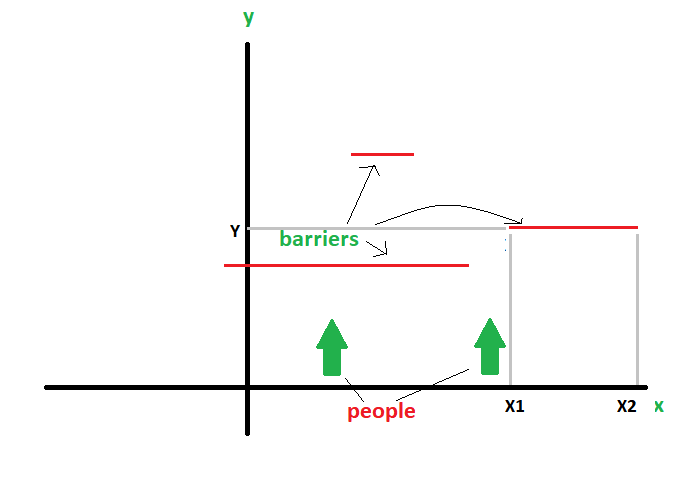# Count points covered by given intervals

• Difficulty Level : Medium
• Last Updated : 29 Sep, 2017

Consider an infinite x-y plane. Infinite people walk on the plane in upward or +ve Y direction. At each integer point on the x-axis, only one person walks. Suppose, several barriers exist parallel to the x-axis. Barriers are provided as three truplets

• X1 – the x-point at which the barrier starts
• X2 – the x-point at which the barrier ends.
• Y – the point on the Y axis where the barrier lies.

Calculate how many people will get stuck at any point due to the barriers. Use of extra space to keep track of different points on x axis is not allowed.Examples:

```Input : barriers[] = {{3 6 2}, {-7 4 3}
Output : 14
The barrier from 3 to 6 blocks 3, 4, 5, 6,
so 4 persons blocked till now.
The barrier from -7 to 4 blocks -7,-6,-5,-4,-
3, -2, -1, 0, 1, 2, 3, 4. But, 3 and 4 have
So, total persons blocked is 14.
```

Asked in: Microsoft IDC Internship.

## Recommended: Please try your approach on {IDE} first, before moving on to the solution.

A simple approach is to use a very long array initialized to zero. Then we can mark those values by 1 which are in the barrier by looping through each barrier. This would solve the case of overlapping of barriers.

But we cannot use another array as mentioned before. Thus, we use sorting and simple math. Following are the steps:

1. Sort all barriers by x1 (Starting point)
2. After sorting, 3 cases arrive:
……I. The next barrier does not overlap with the previous. In this case, we simply add count of points covered by current barrier.
……II. The next barrier partly overlaps the previous one. In this point we add non-overlapping points covered by current barrier.
……III. The next barrier completely overlaps the previous barrier. In this case, we simply ignore current barrier.

Below is the implementation of above approach.

 `// CPP program to find number of people``// that are stopped by barriers``#include ``using` `namespace` `std;`` ` `struct` `Barrier``{``    ``int` `x1, x2, y;``};`` ` `// Compares two Barriers according to x1 ``bool` `compareBarrier(Barrier b1, Barrier b2)``{``    ``return` `(b1.x1 < b2.x1);``}`` ` `// Returns number of people blocked by``// array of barriers arr[0..n-1]``int` `countStopped(Barrier arr[], ``int` `n)``{``    ``// Sort barriers according to x1.``    ``sort(arr, arr+n, compareBarrier);`` ` `    ``// End point of previous barrier``    ``// Initializing with some value``    ``// smaller than lowest x1.``    ``int` `prev_end = arr.x1 - 1;`` ` `    ``// Traverse through all bariers``    ``int` `count = 0;``    ``for` `(``int` `i=0; i

Output :

`14`

We can easily note that there is no importance of y in the question, so it may not be stored.

Time Complexity : O(n Log n)

This article is contributed by Suprotik Dey. If you like GeeksforGeeks and would like to contribute, you can also write an article using contribute.geeksforgeeks.org or mail your article to contribute@geeksforgeeks.org. See your article appearing on the GeeksforGeeks main page and help other Geeks.

Please write comments if you find anything incorrect, or you want to share more information about the topic discussed above.

My Personal Notes arrow_drop_up